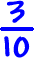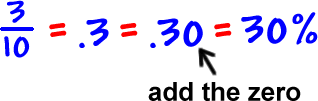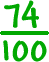Converting a fraction to a percent takes two steps:

 1
Convert the fractions to a decimal.
 2
Convert the decimal to a percent.

If the denominator is 10 or 100, then it's a snap!

 Convertto a percent:Convertto a percent:Convertto a percent:
 Convertto a percent: